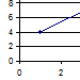Calculating Concurrency from Performance Test Results

So you are on a performance test engagement and your boss asks how many people concurrently executing certain transactions like buying a book or doing a search. He wants is a measure of active concurrency – how many people are doing certain transaction. This should not be confused with Passive concurrency like how many people are logged in. Before we go anyfuther lets clarify that in this example a transaction is a request to the test system and a response back it does not include any think time. Now before you start getting out the virtual terminal server and incrementing counters at the start of the transaction and decrementing counters at the end. There is an easier way.

You can work this all out from your performance test results, without the need for code. Using a mathematical formula (it’s very simple so don’t panic) called Littles Law. Littles Law was first used to analyse the performance of telephone exchanges in 1969 by John Little.
Little’s law allows us to relate the mean number of items in the system in our case concurrent users with the mean time in the system (response time) as follows:

Number of Items in the system = Arrival Rate x Response Time

There is one rule to remember before you use little law you must make sure the system is balanced. That is the arrival rate into the system is the same at the exit rate.

I will begin with a none computer example the “Black Horse Pub” has a mean arrival rate of 5 customers per hour that stay for on average half an hour. Using Little’s law we can calculate the mean number of customers in the pub as Arrival Rate x Response Time = 5 x 0.5 = 2.5 customers.

To apply little law to a performance test we must first make sure that we are taking measurements from when the system under test is balanced. Remember a balanced system the rate of work entering the system matches the rate of work leaving the system. This for a typical load testing tool is after the ramp up period and the number of virtual users remains constant and response times have stabilised and the transaction per second graph is level. To capture this period of time in LoadRunner for example you would need to select the time period in the Summary report filter or under the Tools -> Options.
So record the average response time for the transaction of interest and the number of times per second the transaction is executed.So from the example above the response time is 43.613 seconds. The arrival rate is the number of transactions executed divided by the duration. The duration for this example was a 10 minute period as can be confirm by the LoadRunner summary below.This gives you an arrival rate of 2.005 calculated by taking the count 1203 divided by the duration 600.

So the concurrent number of users waiting for a search to return is 87.44
There you go from your performance test results you can easily calculate the concurrency for a particular transaction.